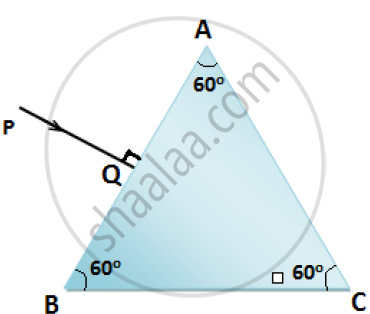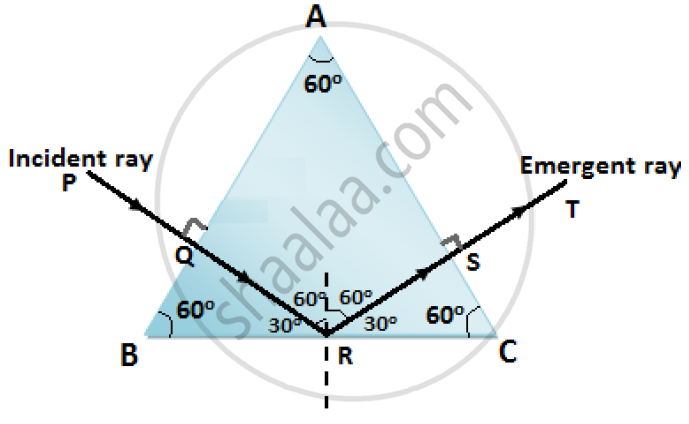Share

# Copy the Diagram Given Below and Complete the Path of the Light Ray Till It Emerges Out of the Prism. the Critical Angle of Glass is 42°. in Your Diagram Mark the Angles Wherever Necessary - ICSE Class 10 - Physics

ConceptRefraction of Light Refraction of Light Through a Glass Block and a Triangular Prism

#### Question

Copy the diagram given below and complete the path of the light ray till it emerges out of the prism. The critical angle of glass is 42°. In your diagram mark the angles wherever necessary.#### SolutionIs there an error in this question or solution?

#### APPEARS IN

Solution Copy the Diagram Given Below and Complete the Path of the Light Ray Till It Emerges Out of the Prism. the Critical Angle of Glass is 42°. in Your Diagram Mark the Angles Wherever Necessary Concept: Refraction of Light - Refraction of Light Through a Glass Block and a Triangular Prism.
S